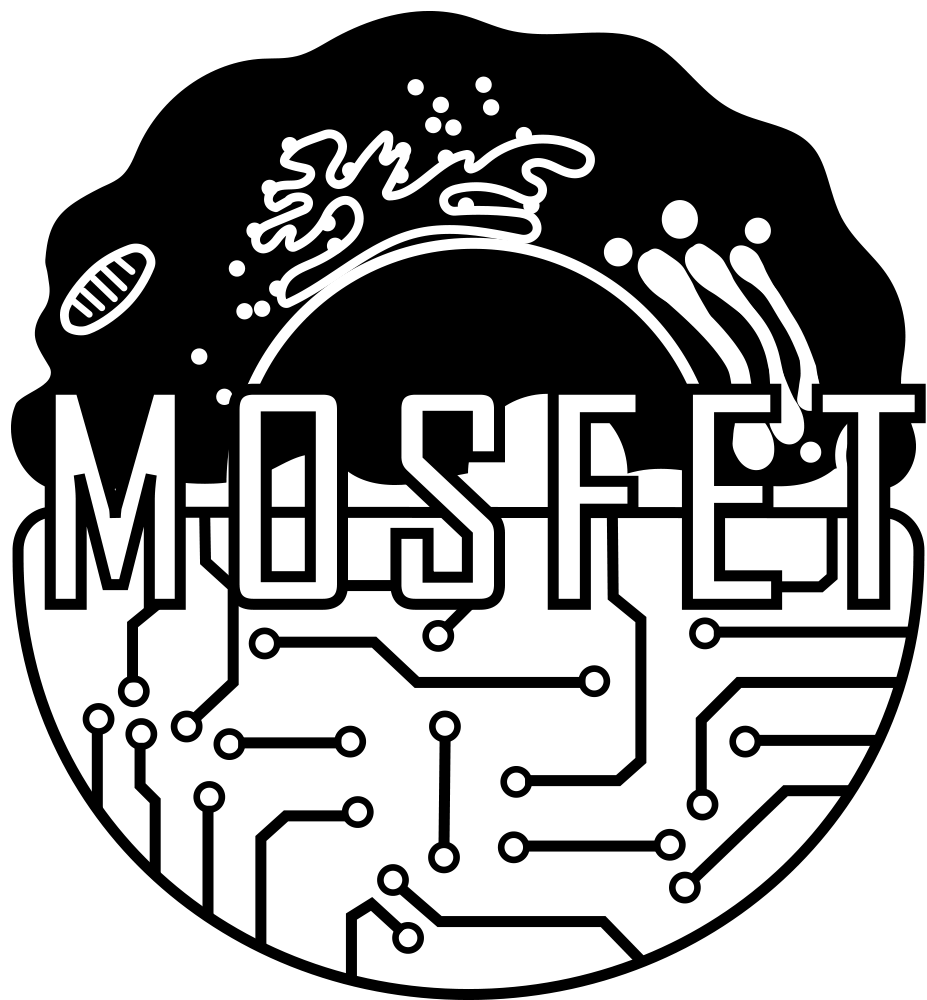# Team:NAU-CHINA/Model

Template:2018_NAU-CHINAEngagement

Model

Overview# Introduction

We propose post-integration conditions, rationally simplify the complex situation, and split the whole process into a series of chemical reactions. Assuming that intervals between two reactions obey exponential distribution, we use the Gillespie algorithm to calculate the changes in various substances in the system with reference to the Dynamics of the Brusselator . The ideas and methods of this model have strong promotion prospects and adaptability. Our model demonstrates the necessity of using the recombinase(rec) system，the improvement effect of the system after adding the pathways expressing RDF-inhibitor and rec-inhibitor in turn, and the robustness of the model .The experimental team verified some assumptions and results of the model and selected materials according to the parameters of the model.

# For judging handbook

What kind of modeling is being done and what information it will provide?

We use the Gillespie algorithm to calculate the changes in various substances in the system

What assumptions were made and why?

One of our assumptions is that the length of the interval between consecutive reactions obeys an exponential distribution so that we can use the Gillespie algorithm  to calculate the changes in various substances in the system.

What kind of data was used to build/assess the model

(a) Expression rate of each gene (production rate of related proteins, consumption rate of each protein.
(b) The computing coefficient of each reaction’s rate.

How the model results affected the project design and development?

Our model shows the improvement effect of the system after adding the pathways expressing RDF-inhibitor and rec-inhibitor in turn, so as to provide guidance to the experimental team.

1. How impressive is the modeling?

Our model has successfully done a proof of concept and played a key role in guiding experimental direction and path design.

2. Did the model help the team understand a part, device, or system?

Of course we did

3. Did the team use measurements of a part, device, or system to develop the model?

The experimental group verifies the feasibility of the path step by step and roughly determines the parameter dimensions of the model.

4. Does the modeling approach provide a good example for others?

The ideas and methods of this model have strong promotion prospects and adaptability. The codes are showed on our wiki , you can copy and run in you Matlab convenient.

# Symbol System

Symbol Meaning
Grec Gene of recombinase
rec Recombinase 
GsynNotch-TEV SynNotch-TEV gene
synNotch Active synNotch on endomembrane system. In our model synNotch is synNotch-TEV
GTetR Gene of operon TetO’s repressor proteins
TetR TetO operon’s repressor proteins
tetO Operon which can be repressed by TetR
TetOR Binary complex of operon TetO and repressor proteins TetR
TEV synNotch’s intracellular domain which is falling off by shearing, an enzyme can divide TetOR binary complex while separate TetR and degrade it.
RDF Reverse recombination factor, which can inverse DNA sequence between sites and make it back to the morphology not affected by rec.
Ts Target signal
Gx Gene of a protein x
U Unconsidered substance

# Assumptions

Tips: Click the button named Aim.

1. Normal protein expression is a reaction which satisfies the post-integration conditions. All reactions which satisfy post-integration conditions can be viewed as one single chemical reaction. part1 . Aim>>To simplify calculation

2. Different reactions in cells occur independently. Aim>>To determine when the next reaction occurs and which reaction occurs.

3. The length of the interval between consecutive reactions obeys an exponential distribution. Aim>>To determine when the next reaction occurs and which reaction occurs.

4. The degradation of protein can be viewed as linear degradation. Aim>>To simplified calculation，this model is also compatible with other methods of calculating degradation for example Michaelis-Menten equation.

5. The repressor effect of the protein can be described by the Hill equation. Aim>>To calculate the expression of rec.

# Parameters

 c21=5; % TetR Gene expression c22=0.001; % TetR degradation c23=200; % generate dipolymer c24=0.003; % TEV causes decomposition of dipolymer c25=0.000005; % self decomposition of dipolymer NTetO=5; % Hill coefficient Swichpoint=2000; % parameter to the hill equation c31=8; % rec's Maximum gene expression rate c32=12; % RDF-inhibitor's Maximum gene expression rate c33=0.01; % rec degradation c34=0.01; % rec-RDF-inhibitor degradation c35=2; % rec-inhibitor Gene expression（No rec-inhibitor can be set to 0） c36=0.1; % rec-inhibitor in combination with rec c37=200; % The noise reduction reaction of rec c41=0.0000006; % rec reverse reaction c51=7; % Turn on GENE expression downstream c52=0.01; % RDF degradation c53=200; % rec-inhibitor in combination with rec c61=0.0000005; % rec-RDF reverse reaction

# References

 Gillespie, D. T. Exact Stochastic Simulation of couple chemical reactions. J. Phys. Chem. 81, 2340–2361 (1977).

 Ault, S. & Holmgreen, E. Dynamics of the Brusselator. Math 715 Proj. (Autumn 2002) 1–17 (2003). doi:10.1103/PhysRevE.61.2361

 Stark WM. 2014. The serine recombinase. MicrobiolSpectrum2 (6):MDNA3-0046-2014.

 Morsut, L. et al. Engineering Customized Cell Sensing and Response Behaviors Using Synthetic Notch Receptors. Cell 164, 780–791 (2016).

 Ramos, J. L. et al. The TetR Family of Transcriptional Repressors The TetR Family of Transcriptional Repressors. Microbiol. Mol. Biol. Rev. 69, 326–356 (2005).

 Phan, J. et al. Structural basis for the substrate specificity of tobacco etch virus protease. J. Biol. Chem. 277, 50564–50572 (2002).

 Olorunniji, F. J. et al. Control of serine integrase recombination directionality by fusion with the directionality factor. Nucleic Acids Res. 45, 8635–8645 (2017).

footer